## Quiz 3 : PercentsLooking for Management Homework Help?# Quiz 3 : Percents

The Percent formula is expressed as,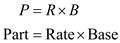The base that is the whole or total is related with the rate which is the number followed by % and part. Complete the following table in the following way: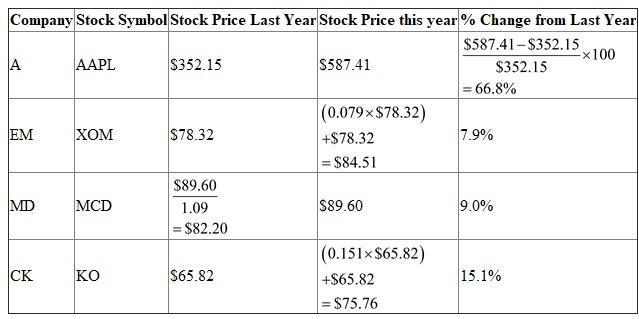The formula of percentage…… (1) D owned a small apartment complex worth \$865,000 last year, and he sold the same for \$892,680 this year. Find out the percent increase in value in the following way: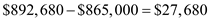The change in amount comes out to be \$27,680 and the base is \$865,000. Use the formula in equation (1) and solve the percentage increase in value.Hence, the percent increase in value is 3.2%.

Recall the property that you can change from a decimal to a percent by moving the decimal point two places to the right and adding a percent sign. You get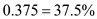In conclusion, you obtain that the percent form for 0.375 is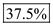.